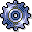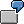Structuring RevaluationsUse

The diagram below shows the criteria according to which you can structure a revaluation. It is possible to revaluate multiple areas of the controlling area according to different aspects.

Defining a Revaluation

 Cost center Cost element All cost elements m Cost element groups All cost centers 1 revaluation m revaluations n sub-hierarchies n revaluations n x m revaluations

If you want to revaluate the entire controlling area based on the same criteria, you require only one revaluation. The following section contains an example of a revaluation:The total primary costs are to be increased by 10% for the shop floor area, and by 5% for the logistics area. You define a separate revaluation for each area.

Use selection criteria to specify the cost center(s) and cost element(s) you want to revalue. Then set the percentage rates. You can choose between:

• Revaluation of costs
• Revaluation of consumption